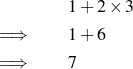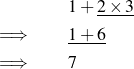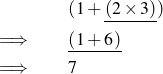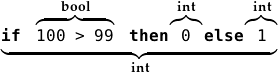Back to Contents

# Starting Off

We will cover a fair amount of material in this chapter and its questions, since we will need a solid base on which to build. You should read this with a computer running OCaml in front of you.

Consider first the mathematical expression 1 + 2 × 3. What is the result? How did you work it out? We might show the process like this:How did we know to multiply 2 by 3 first, instead of adding 1 and 2? How did we know when to stop? Let us underline the part of the expression which is dealt with at each step:We chose which part of the expression to deal with each time using a familiar mathematical rule – multiplication is done before addition. We stopped when the expression could not be processed any further.

Computer programs in OCaml are just like these expressions. In order to give you an answer, the computer needs to know all the rules you know about how to process the expression correctly. In fact, 1 + 2 × 3 is a valid OCaml expression as well as a valid mathematical one, but we must write `*` instead of ×, since there is no × key on the keyboard:

` OCaml`

`# 1 + 2 * 3;;`
`- : int = 7`

Here, `#` is OCaml prompting us to write an expression, and `1 + 2 * 3;;` is what we typed (the semicolons followed by the Enter key tell OCaml we have finished our expression). OCaml responds with the answer `7`. OCaml also prints `int`, which tells us that the answer is a whole number, or integer.

Let us look at our example expression some more. There are two operators: + and ×. There are three operands: 1, 2, and 3. When we wrote it down, and when we typed it into OCaml, we put spaces between the operators and operands for readability. How does OCaml process it? Firstly, the text we wrote must be split up into its basic parts: `1`, `+`, `2`, `*`, and `3`. OCaml then looks at the order and kind of the operators and operands, and decides how to parenthesize the expression: (1+(2×3)). Now, processing the expression just requires dealing with each parenthesized section, starting with the innermost, and stopping when there are no parentheses left:OCaml knows that × is to be done before +, and parenthesizes the expression appropriately. We say the × operator has higher precedence than the + operator.

An expression is any valid OCaml program. To produce an answer, OCaml evaluates the expression, yielding a special kind of expression, a value. In our previous example, 1 + 2 × 3, 1 + 6, and 7 were all expressions, but only 7 was a value.

Each expression (and so each value) has a type. The type of 7 is int (it is an integer). The types of the expressions 1 + 6 and 1 + 2 × 3 are also int, since they will evaluate to a value of type int. The type of any expression may be worked out by considering the types of its sub-expressions, and how they are combined to form the expression. For example, 1 + 6 has type int because 1 is an int, 6 is an int, and the + operator takes two integers and gives another one (their sum). Here are the mathematical operators on integers:

Operator Description
a `+` b addition
a `-` b subtract b from a
a `*` b multiplication
a `/` b divide a by b, returning the whole part
a `mod` b divide a by b, returning the remainder

The `mod`, `*`, and `/` operators have higher precedence than the `+` and `-` operators. For any operator above, the expression a ⊕ b ⊕ c is equivalent to (a ⊕ b) ⊕ c rather than a ⊕ (b ⊕ c) (we say the operators are left associative). We sometimes write down the type of an expression after a colon when working on paper, to keep it in mind:

`5 * -2 :` int

(negative numbers are written with `-` before them). Of course, there are many more types than just int. Sometimes, instead of numbers, we would like to talk about truth: either something is true or it is not. For this we use the type bool which represents boolean values, named after the English mathematician George Boole (1815–1864) who pioneered their use. There are just two things of type bool:

`true`
`false`

How can we use these? One way is to use one of the comparison operators, which are used for comparing values to one another. For example:

` OCaml`

`# 99 > 100;;`
`- : bool = false`
`# 4 + 3 + 2 + 1 = 10;;`
`- : bool = true`

Here are all the comparison operators:

Operator Description
a `=` b true if a and b are equal
a `<` b true if a is less than b
a `<=` b true if a is less than or equal to b
a `>` b true if a is more than b
a `>=` b true if a is more than or equal to b
a `<>` b true if a is not equal to b

Notice that if we try to use operators with types for which they are not intended, OCaml will not accept the program at all, showing us where our mistake is by underlining it:

` OCaml`

`# 1 + ``true``;;`
`Error: This expression has type bool but an expression was expected of type`
` int`

You can find more information about error messages in OCaml in the appendix “Coping with Errors” at the back of this book.

There are two operators for combining boolean values (for instance, those resulting from using the comparison operators). The expression `a && b` evaluates to `true` only if expressions a and b both evaluate to `true`. The expression `a || b` evaluates to `true` only if a evaluates to `true`, b evaluates to `true`, or both do. The `&&` operator (pronounced “and”) is of higher precedence than the `||` operator (pronounced “or”), so `a && b || c` is the same as `(a && b) || c`.

A third type we shall be using is char which holds a single character, such as ‘a’ or ‘?’. We write these in single quotation marks:

` OCaml`

`# ’c’;;`
`- : char = ’c’`

So far we have looked only at operators like `+`, `mod`, and `=` which look like familiar mathematical ones. But many constructs in programming languages look a little different. For example, to choose a course of evaluation based on some test, we use the `if` … `then` … `else` construct:

` OCaml`

`# if 100 > 99 then 0 else 1;;`
`- : int = 0`

The expression between `if` and `then` (in our example `100 > 99`) must have type bool – it evaluates to either `true` or `false`. The types of the expression to choose if true and the expression to choose if false must be the same as one another – here they are both of type int. The whole expression evaluates to the same type – int – because either the `then` part or the `else` part is chosen to be the result of evaluating the whole expression:We have covered a lot in this chapter, but we need all these basic tools before we can write interesting programs. Make sure you work through the questions on paper, on the computer, or both, before moving on. Hints and answers are at the back of the book.

## Questions

1. What are the types of the following expressions and what do they evaluate to, and why?

`17`

`1 + 2 * 3 + 4`

`800 / 80 / 8`

`400 > 200`

`1 <> 1`

`true || false`

`true && false`

`if`` true ``then`` false ``else`` true`

`’%’`

`’a’ + ’b’`

2. Consider the evaluations of the expressions `1 + 2 mod 3`, `(1 + 2) mod 3`, and `1 + (2 mod 3)`. What can you conclude about the `+` and `mod` operators?

3. A programmer writes `1+2 * 3+4`. What does this evaluate to? What advice would you give him?

4. The range of numbers available is limited. There are two special numbers: `min_int` and `max_int`. What are their values on your computer? What happens when you evaluate the expressions `max_int + 1` and `min_int - 1`?

5. What happens when you try to evaluate the expression `1 / 0`? Why?

6. Can you discover what the `mod` operator does when one or both of the operands are negative? What about if the first operand is zero? What if the second is zero?

7. Why not just use, for example, the integer `0` to represent false and the integer `1` for true? Why have a separate bool type at all?

8. What is the effect of the comparison operators like `<` and `>` on alphabetic values of type char? For example, what does `’p’ < ’q’` evaluate to? What is the effect of the comparison operators on the booleans, `true` and `false`?